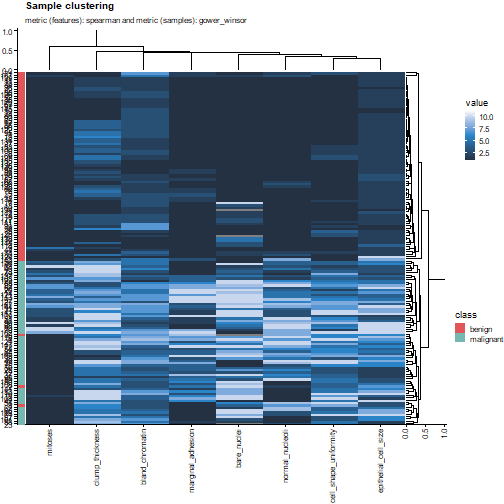# Evaluation and explanation

#### 2022-08-12

library(familiar)
library(data.table)

set.seed(19)

Familiar will automatically evaluate created models, and export corresponding tables and plots. The table below lists all available analyses. Several analyses allow for determining distributions and confidence intervals to provide an indication of the value spread for a given dataset and models (if applicable). The estimation_type argument defines this behaviour:

• point: Compute point estimates, i.e. single values.

• bias_correction or bc: Bias-corrected estimates. A bias-corrected estimate is computed from (at least) 20 point estimates.

• bootstrap_confidence_interval or bci: Bias-corrected estimates with bootstrap confidence intervals (Efron and Hastie 2016). This estimation type allows for plotting confidence intervals in plots. The number of point estimates required depends on the confidence_level parameter. Familiar uses a rule of thumb of $$n=20/(1-\textrm{confidence_level})$$, i.e. at least 400 points for confidence_level=0.95.

Another argument, detail_level determines how the required point estimates are formed:

• ensemble: Point estimates are computed at the ensemble level, i.e. over all models in the ensemble. This means that, for example, bias-corrected estimates of model performance are assessed by creating (at least) 20 bootstraps and computing the model performance of the ensemble model for each bootstrap.

• hybrid: Point estimates are computed from the models in an ensemble. This means that, for example, bias-corrected estimates of model performance are directly computed using the models in the ensemble. If there are at least 20 trained models in the ensemble, performance is computed for each model, in contrast to ensemble where performance is computed for the ensemble of models. If there are less than 20 trained models in the ensemble, bootstraps are created so that at least 20 point estimates can be made.

• model: Point estimates are computed at the model level. This means that, for example, bias-corrected estimates of model performance are assessed by creating (at least) 20 bootstraps and computing the performance of the model for each bootstrap. Familiar will not automatically create plots in this case.

The more point estimates are required, the more computationally intensive the analysis is. Some analyses also become computationally more expensive with more samples. The sample_limit argument can be used to specify the maximum number of samples that should be used.

Some analyses, such as model predictions and individual conditional expectation plots, do not benefit from bootstraps as they are solely based on values predicted by the (ensemble) models. If you want to compute bias-corrected estimates or bootstrap confidence intervals for these analyses you should ensure that sufficient models are created. Experimental designs such as experiment_design="bs(fs+mb,400)+ev" allow for this, but are computationally expensive.

Evaluation and explanation steps.
a Available for binomial and multinomial outcomes.
b Available for survival outcomes.
c Available for all outcomes.
d Estimation types other than point require sufficient models.
e May not be available for all models.
Name Plot function Export function Estimation type Detail level Sample limit
AUC-PRa plot_auc_precision_recall_curve export_auc_data × ×
AUC-ROCa plot_auc_roc_curve export_auc_data × ×
Calibration infoc export_calibration_info ×
Confusion matrixa plot_confusion_matrix export_confusion_matrix_data ×
Decision curve analysisab plot_decision_curve export_decision_curve_analysis_data × ×
Feature expressionc plot_sample_clustering export_feature_expressions
Feature selection variable importancec plot_feature_selection_occurrence; plot_feature_selection_variable_importance export_fs_vimp
Feature similarityc plot_feature_similarity export_feature_similarity ×
Hyperparametersc export_hyperparameters
Individual conditional expectationc plot_ice export_ice_data ×d × ×
Model calibrationce plot_calibration_data export_calibration_data × ×
Model performancec plot_model_performance export_model_performance × ×
Model predictionsc export_prediction_data ×d ×
Model variable importancece plot_model_signature_occurrence; plot_model_signature_variable_importance export_model_vimp
Partial dependencec plot_pd export_partial_dependence_data ×d × ×
Permutation variable importancec plot_permutation_variable_importance export_permutation_vimp × ×
Risk stratification infob export_risk_stratification_info ×
Risk stratification datab plot_kaplan_meier export_risk_stratification_data ×
Sample similarityc export_sample_similarity × ×
Univariate analysisc plot_univariate_importance export_univariate_analysis_data

When familiar is run from summon_familiar plots and tables are exported automatically. The corresponding plot and export functions can all be used externally as well, as is described in the Using familiar prospectively vignette. Indeed, to showcase some of the functionality we will employ the techniques discussed there.

We will first create two models. We train the first model using (a subset of) the Wisconsin breast cancer dataset (Wolberg and Mangasarian 1990) to predict cell malignancy from biopsies, which constitutes a classification problem.


# Get a dataset.
binomial_data <- familiar:::test.create_good_data_set(outcome_type="binomial")

# Call familiar to create models. Note that we skip hyperparameter optimisation by manually defining the hyperparameters, and that we skip evaluation steps. This is to save time when creating the vignette.
familiar::summon_familiar(data=binomial_data,
project_dir=file.path(tempdir()),
experiment_dir="binomial",
experimental_design="fs+mb",
cluster_method="none",
fs_method="none",
hyperparameter=list("random_forest_ranger"=
list("n_tree"=4,
"sample_size"=0.7,
"m_try"=0.5,
"node_size"=20,
"tree_depth"=3)),
learner="random_forest_ranger",
parallel=FALSE,
skip_evaluation_elements="all")

# Create path to the directory containing the models.
model_directory_path <- file.path(tempdir(), "binomial", "trained_models", "random_forest_ranger", "none")
model_path <- file.path(model_directory_path, list.files(model_directory_path, pattern="model"))

binomial_model <- readRDS(model_path)

The second model is a survival model, and is trained on (a subset of) a colon chemotherapy clinical trial dataset (Moertel et al. 1995) to predict overall survival after treatment.


# Get a dataset.
survival_data <- familiar:::test.create_good_data_set(outcome_type="survival")

# Call familiar to create models. Note that we skip hyperparameter optimisation by manually defining the hyperparameters, and that we skip evaluation steps. This is to save time when creating the vignette.
familiar::summon_familiar(data=survival_data,
project_dir=file.path(tempdir()),
experiment_dir="survival",
experimental_design="fs+mb",
cluster_method="none",
fs_method="none",
hyperparameter=list("random_forest_ranger"=
list("n_tree"=9,
"sample_size"=0.95,
"m_try"=0.68,
"node_size"=30,
"tree_depth"=2,
"alpha"=0.25)),
learner="random_forest_ranger",
parallel=FALSE,
skip_evaluation_elements="all")

# Create path to the directory containing the models.
model_directory_path <- file.path(tempdir(), "survival", "trained_models", "random_forest_ranger", "none")
model_path <- file.path(model_directory_path, list.files(model_directory_path, pattern="model"))

survival_model <- readRDS(model_path)

# Model performance

We assess model performance to evaluate accuracy of a model. Model accuracy is quantified using performance metrics that are documented in the Performance metrics vignette, and can be set using the evaluation_metric argument of the summon_familiar function or metric for plot and export functions. For example, this results in the following plot for the breast cancer dataset:

plots <- familiar::plot_model_performance(object=binomial_model,
data=binomial_data,
metric = c("auc", "brier", "f1_score"),
x_axis_by="metric")

plots[]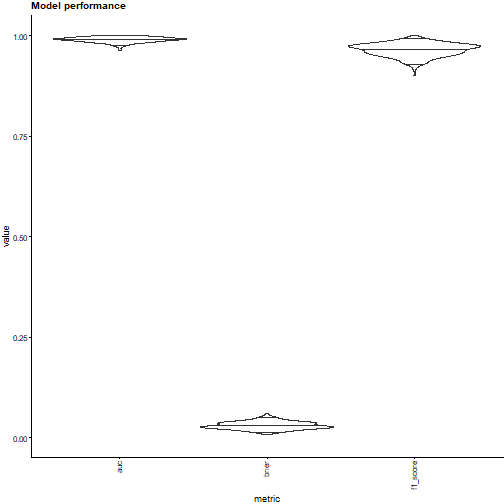The plot shows distributions of the area under the receiver operating characteristic curve, the brier score and the f1-score. The plot indicates that the model predicts accurately for the given dataset. This behaviour is expected: The classification problem is quite easy, and we are evaluating the development dataset.

To compute model performance metric scores for a dataset, familiar first predicts expected values for instances in a dataset. Familiar then compares the expected values against the observed values, and computes the value of the specified metric.

The receiver-operating characteristic (ROC) curve visualises concordance between predicted class probabilities and the observed classes (Hand and Till 2001). It shows the trade-off between sensitivity and specificity of the model. The receiver-operating characteristic curve for the breast cancer dataset is as follows:

plots <- familiar::plot_auc_roc_curve(object=binomial_model,
data=binomial_data)

plots[]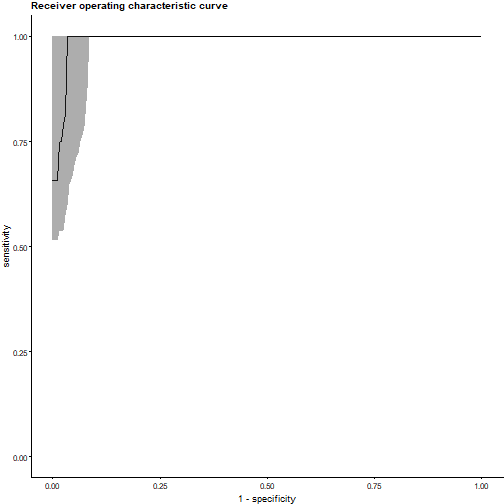Under ideal circumstances, the curve would lie in the top-left corner of the plot. If a model does not predict better than at random, the curve would lie along the diagonal, which corresponds to an AUC-ROC value of 0.50. The plot above indicates that the model can differentiate between benign and malignant cells in breast cancer biopsy.

Familiar creates an ROC curve by ascendingly sorting instances by the predicted probability of the positive class, and computing the number of true positive, true negative, false positive, false negative cases observed at each instance. These are then used to compute sensitivity and specificity.

The above plot shows the average of 400 single curves, with 95% confidence intervals. To achieve this, familiar interrogates each curve over the complete range of potential specificity values ($$[0.000, 1.000]$$) with a step size of $$0.005$$. Linear interpolation is used for this purpose (Davis and Goadrich 2006). This allows for assessing the distribution of sensitivity at fixed specificity values.

The above approach is the general approach used by familiar. However, familiar will plot an exact ROC curve if the point estimate (estimation_type="point") of a single model or the complete ensemble of models is evaluated (detail_level="ensemble"):

plots <- familiar::plot_auc_roc_curve(object=binomial_model,
data=binomial_data,
estimation_type="point")

plots[]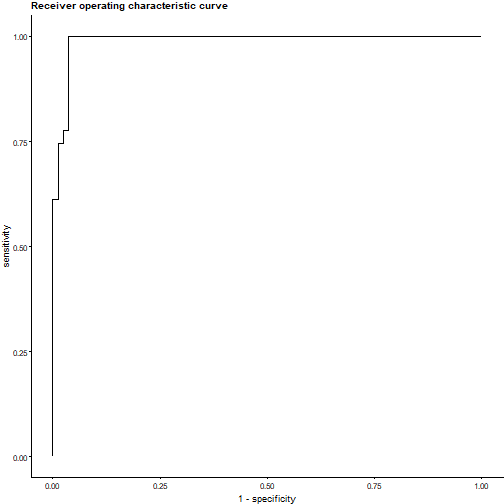Note that we did not specify the detail level to create the above plot because only a single model is evaluated.

## Precision-recall curve

The precision-recall (PR) curve shows the trade-off between precision (positive predictive value) and recall (sensitivity). An important distinction between the PR curve and the ROC curve described above is that precision does not assess true negatives but false positives. In case the number of negative instances greatly exceeds the number of positive instances, a large change in the number of false positives reduces the ROC curve somewhat, but this change is far easier observed in the PR curve (Davis and Goadrich 2006). Hence the PR curve is commonly assessed if the positive class is more important than and rare compared to the negative class. For the breast cancer dataset, the precision-recall curve is as follows:

plots <- familiar::plot_auc_precision_recall_curve(object=binomial_model,
data=binomial_data)

plots[]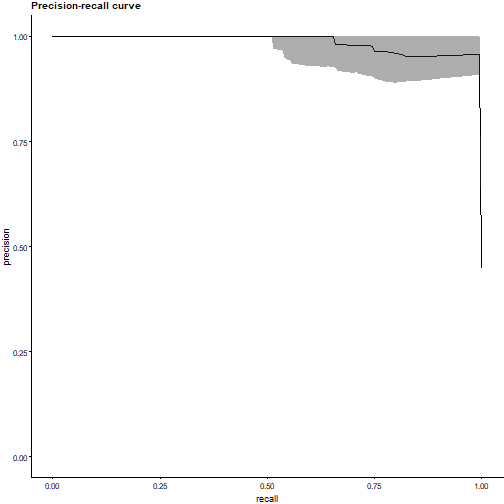Under ideal circumstances, the curve would lie in the top-right part of the plot. A random classifier would yield a curve that is mostly horizontal and located at the fraction of positive instances. This would equal a precision of 45% for the current dataset.

Like the ROC curve, familiar forms the PR curve by ascendingly sorting instances by the predicted probability of the positive class and computing the number of true positive and false positive negative cases observed at each instance. These values are then used to compute recall and precision.

The above plot shows the average of 400 single curves, with 95% confidence intervals. To achieve this, familiar interrogates each curve over the complete range of recall values ($$[0.000, 1.000]$$) with a step size of $$0.005$$. We use linear interpolation for this purpose, treating the PR curve as a piecewise function to manage the situation identified by Davis and Goadrich (Davis and Goadrich 2006). Interpolating in this manner allows for assessing the distribution of precision values at fixed recall values.

Again, this is the general approach used by familiar. Familiar can plot an exact PR curve when evaluating the point estimate (estimation_type="point") of a single model or of the complete ensemble of models (detail_level="ensemble"). In the breast cancer dataset, this produces the following curve:

plots <- familiar::plot_auc_precision_recall_curve(object=binomial_model,
data=binomial_data,
estimation_type="point")

plots[]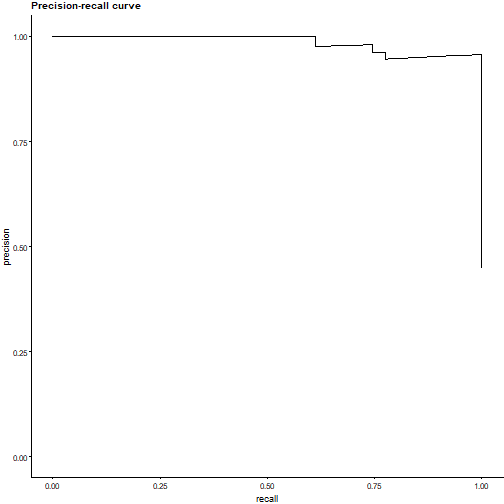## Confusion matrix

A confusion matrix schematically represents the number of predicted (expected) class instances and their actual (observed) values. This may help identify potential weaknesses of classifiers, i.e. false positives or negatives.

plots <- familiar::plot_confusion_matrix(object=binomial_model,
data=binomial_data)

plots[]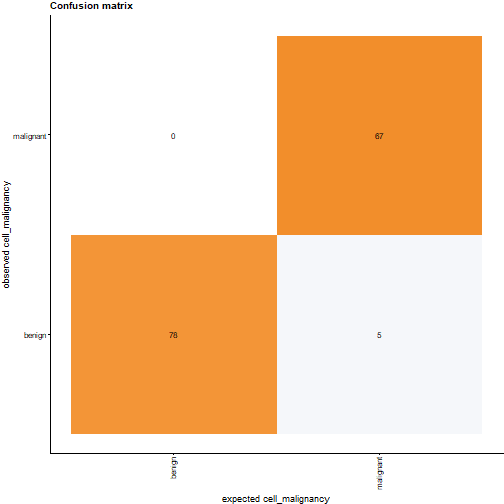Familiar selects the class with the highest predicted class probability as the expected class. It is currently not possible in familiar to obtain confusion matrices for set probability thresholds, such as the threshold that maximises the Matthews correlation coefficient.

## Kaplan-Meier survival curves

Kaplan-Meier survival curves are a standard method for assessing survival over time in a population, or for assessing survival differences between groups.

Familiar applies one or more thresholds to stratify instances to risk groups. These thresholds are created during model development to avoid bias. The number and value of these thresholds is determined by two parameters: stratification_method and stratification_threshold. Both parameters are set when calling summon_familiar. stratification_method has three options:

• median: Familiar uses the median predicted value in the development cohort to stratify instances into two risk groups.

• fixed: Instances are stratified based on the sample quantiles of the predicted values. These quantiles are defined using the stratification_threshold parameter.

• optimised: Use maximally selected rank statistics to determine the optimal threshold (Lausen and Schumacher 1992; Hothorn and Lausen 2003) to stratify instances into two optimally separated risk groups.

The stratification_threshold parameter is only used when stratification_method="fixed". It allows for specifying the sample quantiles that are used to determine the thresholds. For example stratification_threshold=c(0.25,0.75) creates two thresholds that stratifies the development dataset into three groups: one with 25% of the instances with the highest risk, a group with 50% of the instances with medium risk, and a third group with 25% of instances with lowest risk.

Applying the survival model to the colon cancer dataset yields the following Kaplan-Meier plot:

plots <- familiar::plot_kaplan_meier(object=survival_model,
data=survival_data)

grid::grid.newpage()
grid::grid.draw(plots[])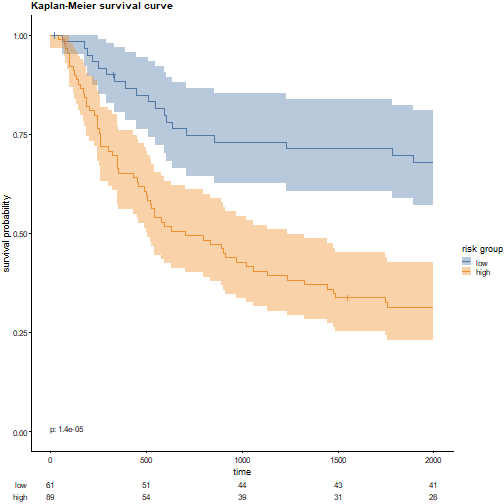In the plot above, familiar divides instances into two groups by the median threshold in the development dataset. The risk groups are shown with 95% confidence intervals. Familiar uses the survival::survfit function to create both the survival curves and the confidence intervals. The low-risk group has significantly better survival than the high-risk group. This is also indicated in the bottom-left of the main plot, which shows the result of a logrank test between the two groups. Crosses indicate right-censored patients, of which there were few. The number of surviving patients in both groups at the time points along the x-axis are shown below the main plot.

Note that the predict method allows for manually specifying stratification thresholds for existing models.

# Model calibration

Evaluating model performance is important as it allows us to assess how accurate a model is. However, it often does not tell us how well we can rely on predictions for individual instances, e.g. how accurate an instance class probability is. Model calibration is assessed for this purpose. The model for the breast cancer dataset produces the following calibration plot:

plots <- familiar::plot_calibration_data(object=binomial_model,
data=binomial_data)

grid::grid.newpage()
grid::grid.draw(plots[])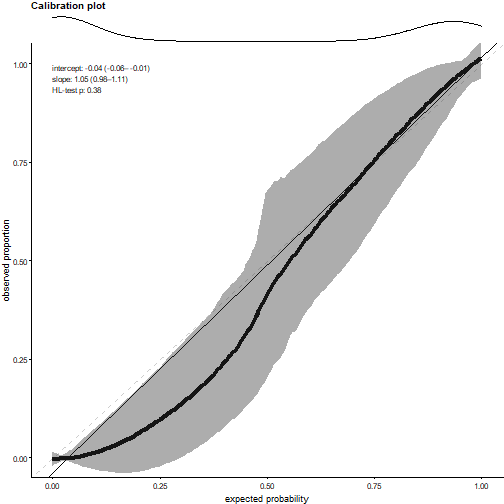The calibration curve shows the expected (predicted) probability of malignant cells versus the observed proportion of malignant cells, together with a 95% confidence interval. In an ideal calibration plot the calibration curve should lie on the diagonal (dashed) line. Here the upper part of the curve (expected probability > 0.50) follows the diagonal nicely. The lower part of the curve dips below the diagonal, indicating that predicted probabilities in this range tend to overestimate malignancy. However, the density plot in the top panel indicates that predicted probabilities were generally close to 0.0 or 1.0, with very few instances in between.

Familiar also performs several calibration tests to assess various degrees of calibration (Van Calster et al. 2016). First of all, we assess two aspects of the linear fit (solid line in the plot): the intercept (calibration-in-the-large) and slope. These are shown in the top-left of the main plot.

The intercept ideally is 0.00. In our example, it lies below this point, and is not contained in the 95% confidence interval. We therefore conclude that the intercept is significantly different at the $$\alpha=0.05$$ level. Note that this difference may not be relevant in practice, as it is quite small.

In an ideal case, the slope of the linear fit is 1.00. In our example, the slope of the linear fit lies above this value. This indicates that, overall, the model tends to overestimate the probability of malignant cells, but not to a large degree.

One issue with assessing calibration through a linear fit, is that the calibration curve can be decidedly non-linear and still produce good values for the intercept and slope. Therefore, we also assess calibration using statistical goodness-of-fit tests: the Hosmer-Lemeshow (HL) test for categorical and regression outcomes (Hosmer et al. 1997), and the Nam-D’Agostino (ND) (D’Agostino and Nam 2003) and Greenwood-Nam-D’Agostino (GND) (Demler, Paynter, and Cook 2015) tests for survival outcomes.

## Implementation details

Here we detail the implementation of the model calibration in familiar. The statistical tests mentioned above, as well as the linear fit, rely on grouping instances. First we order the instances in the dataset by their expected (predicted) values. Within each group, the expected values and observed values are compared:

• Categorical outcomes: We compare the fraction instances with the (positive) observed class in the group against the mean expected probability for that same class.

• Regression outcomes: Both expected and observed values are first normalised to a $$[0, 1]$$ range using the value range observed in the development dataset. We then compare the mean observed value in the group against the mean expected value.

• Survival outcomes: We predict survival probabilities at time points indicated by evaluation_times. The mean expected survival probability in the group is then compared against the observed probability (Kaplan-Meier estimator) at the same time points.

We can observe the points thus created in a calibration plot when estimation_type="point":

plots <- familiar::plot_calibration_data(object=binomial_model,
data=binomial_data,
estimation_type="point")

grid::grid.newpage()
grid::grid.draw(plots[])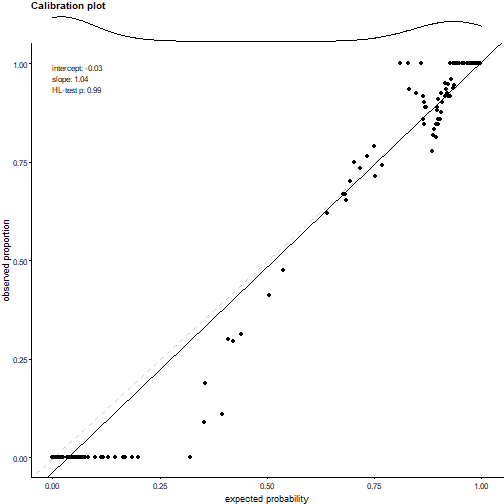The plot with point estimates and the earlier calibration plot with bootstrap confidence intervals lead to the same assessment. However, you may have observed that the number of points, and thus the number of groups, is certainly larger than the 10 groups commonly formed for assessing calibration. This is correct.

Familiar introduces randomness into the assessment of calibration. First, familiar randomises the number of groups drawn because using a fixed number of 10 groups is arbitrary. Second, the actual group sizes are randomised by drawing group identifiers with replacement for all instances. We then sort the set of drawn identifiers, and the instances, ordered by increasing expected value, are then assigned to their respective group. Thirdly, this process is repeated multiple times, i.e. 20 times for point estimates, again 20 times (on bootstraps) for bias-corrected estimates and more for bootstraps confidence intervals.

Linear fit intercept and slope as well as the goodness-of-fit test p-value are determined for each single set of groups. Intercept and slopes are then averaged (point and bias_correction) or assessed as a distribution (bootstrap_confidence_interval). p-values from goodness-of-fit tests need to be combined. These tests are not fully independent because they derive from the same dataset. We therefore compute a harmonic mean p-value (Wilson 2019) from the individual p-values.

# Decision curve analysis

Decision curve analysis is a method to assess the net (clinical) benefit of models, introduced by Vickers and Elkin (Vickers and Elkin 2006). The decision curve compares the model benefit for different threshold probabilities against two or more options. The two standard options are that all instances receive an intervention, and none receive an intervention. What constitutes an intervention is model-dependent (Vickers, Calster, and Steyerberg 2019). For our breast cancer cohort, intervention might constitute treatment of all patients, versus no treatment and treatment for patients with malignancy predicted by the model. This produces the following figure:

plots <- familiar::plot_decision_curve(object=binomial_model,
data=binomial_data,
y_range=c(-0.2, 0.5))

plots[]
#> Warning: Removed 1 row(s) containing missing values (geom_path).
#> Removed 1 row(s) containing missing values (geom_path).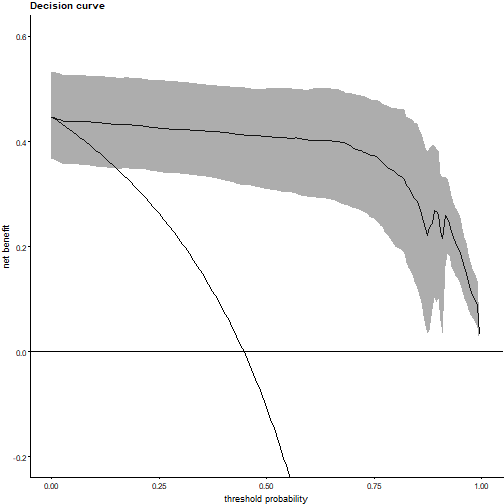The shaded curve is the decision curve produced by the model, with 95% confidence intervals. The declining curve represents the intervention for all, which offers no benefit for a malignancy probability of 45%. The horizontal line at a net benefit of 0.0 represent the no intervention option. As may be observed, the model offers a net benefit over the entire range of threshold probabilities.

For regression problems no definition of decision curves exists. However, familiar can perform decision curve analysis for survival outcomes, based on Vickers et al. (Vickers et al. 2008).

# Variable importance

Not all features are strongly associated with the outcome of interest. One of the most critical points in machine learning is selecting those features that are important enough to incorporate into a model. Familiar prospectively assesses variable importance during the feature selection step using the methods detailed in the Feature selection methods vignette. Later, during evaluation, familiar employs both model-specific and model-agnostic methods to explaining which features are considered important by the model itself.

## Model-specific methods

Model-specific methods rely on aspects of the models themselves to determine variable importance. For instance, the coefficients of regression models are a measure of variable importance when features are normalised. In the case of the random forest model for the breast cancer dataset, permutation variable importance is determined. This produces the following plot:

plots <- familiar::plot_model_signature_variable_importance(object=binomial_model,
data=binomial_data)

plots[]
#> Warning: Removed 1 rows containing missing values (position_stack).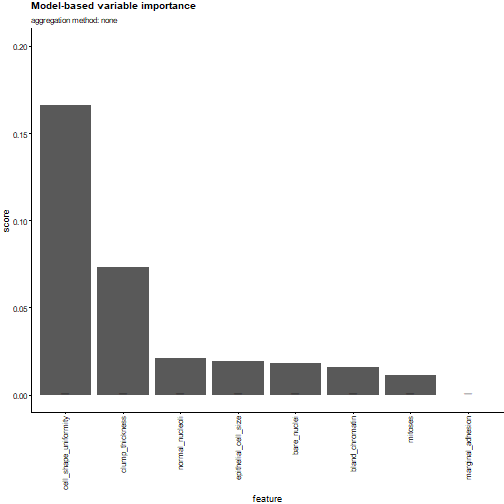In such plots, we always order features so that the most important features are on the left, and the least important features on the right.

In case an ensemble of models is assessed, scores are aggregated using an aggregation method (see the feature selection methods vignette). This method can be set using the aggregation_method argument, or the eval_aggregation_method configuration parameter (summon_familiar).

The plot_model_signature_occurrence method plots the occurrence of features among the first 5 ranks across an ensemble of models (if available). The rank threshold can be specified using the rank_threshold argument or the eval_aggregation_rank_threshold parameter (summon_familiar).

## Permutation variable importance

Permutation variable importance is a model-agnostic variable importance method. It assesses importance by measuring the decrease in model performance caused by shuffling values of a feature across the instances in a dataset.

For the breast cancer dataset, this results in the following figure:

plots <- familiar::plot_permutation_variable_importance(object=binomial_model,
data=binomial_data,
estimation_type="bias_correction")

plots[]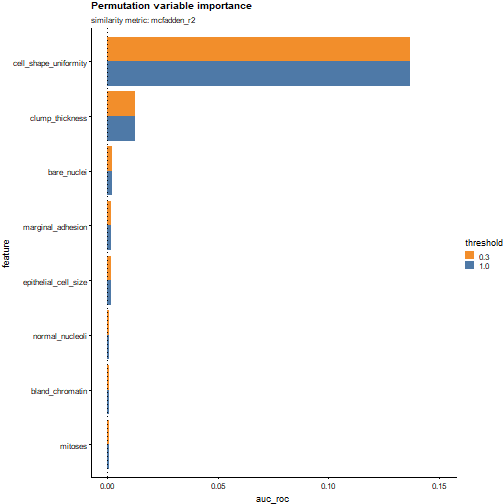The figure shows the features along the y-axis, and the loss in AUC-ROC caused by permuting the feature values along the x-axis. Accordingly, cell_shape_uniformity is considered to be the most important feature in the model.

The figure shows two bars for each feature. A well-known issue with permutation variable importance is that the presence of highly correlated features causes permutation variable importance to become unreliable (Hooker, Mentch, and Zhou 2019). If two features are both important and highly correlated, shuffling one of them does not decrease model performance as much. For this reason, familiar will permute features together depending on their mutual correlation, and the provided threshold values.

By default, similarity between features is assessed using McFadden’s pseudo-$$R^2$$. This metric can assess similarity between different types of features, i.e. numeric and categorical. The default thresholds are 0.3 and 1.0 that respectively cluster highly similar features and permute all features individually.

The breast cancer dataset doesn’t contain features that exceed the relatively stringent threshold of 0.3. Since the breast cancer dataset has only numerical features, we can also measure similarity differently. For example, if we measure similarity using the Spearmans rank correlation coefficient, we arrive at the following:

plots <- familiar::plot_permutation_variable_importance(object=binomial_model,
data=binomial_data,
estimation_type="bias_correction",
feature_similarity_metric="spearman",
feature_similarity_threshold=c(0.6, 1.0))

plots[]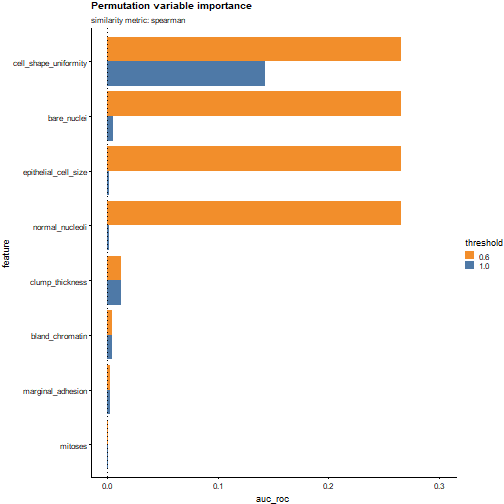This figure paints a different picture: the cell_shape_uniformity, bare_nuclei, normal_nucleoli and epithelial_cell_size features are moderately to strongly correlated. When permuted together, this has a major effect on model performance. This can also be observed in the performance decrease caused by shuffling cell_shape_uniformity alone, which only partially matches the performance decrease caused by shuffling the 4 features. The other 3 features have little individual impact on performance, yet shuffling them together directly shows that these features are important as well.

# Partial dependence and individual conditional expectation plots

Variable importance does not explain how features influence the model. Partial dependence (PD) (Friedman 2001) and individual conditional expectation (ICE) (Goldstein et al. 2015) plots can be used to visualise this behaviour. As an example, we can create an ICE plot for the cell_shape_uniformity feature that was found to be important for predicting cell malignancy in the breast cancer.

plots <- familiar::plot_ice(object=binomial_model,
data=binomial_data,
features="cell_shape_uniformity")

grid::grid.newpage()
grid::grid.draw(plots[])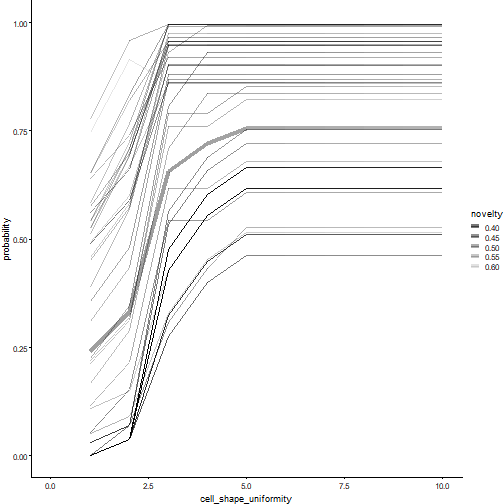The ICE plot shows multiple curves for individual samples, as well as their average, the partial dependence plot as a somewhat thicker curve. The ICE plot indicates that an increase in cell shape uniformity is associated by the model with a rise in malignancy.

It also shows novelty, which is a measure of how out-of-distribution the value is. Novelty is computed using extended isolation forests (Hariri, Carrasco Kind, and Brunner 2019) and is the average normalised height at which a sample is found in a terminal leaf node of the trees in the isolation forest (Liu, Ting, and Zhou 2008). Novelty values range between 0.00 and 1.00. Novelty values should be interpreted with care. While increasing novelty values represent instances that lie further from the distribution, the values that represent in-distribution instances may vary. In the ICE plot above, novelty values range between 0.40 and 0.60. In fact, the median novelty value for the training instances is 0.47 with an interquartile range of 0.07. None of the points in ICE plot can therefore be considered out-of-distribution.

It is possible to anchor the curves in the ICE plot to a specific feature value. This allows for assessing the model response for individual instances versus a fixed value. In effect this can reduce some of the offset caused by other (fixed) features in each instance, and may help to elucidate the observed behaviour:

plots <- familiar::plot_ice(object=binomial_model,
data=binomial_data,
features="cell_shape_uniformity",
anchor_values=list("cell_shape_uniformity"=10.0))

grid::grid.newpage()
grid::grid.draw(plots[])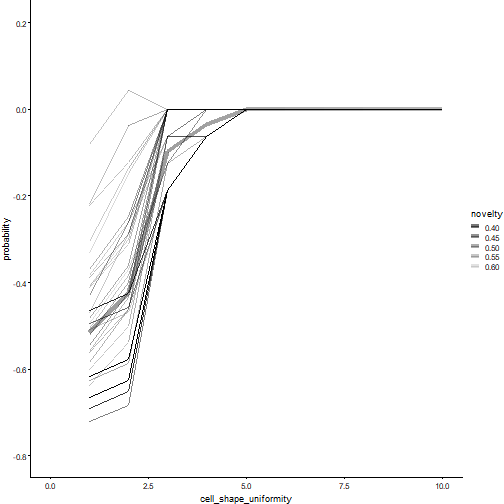In the plot above, we have anchored the values at cell_shape_uniformity=10, which is the maximum value that this feature can take. All curves are then drawn relative to the predicted probability found for this particular value.

It is also possible to show the partial dependence of two features at the same time:

plots <- familiar::plot_ice(object=binomial_model,
data=binomial_data,
features=c("cell_shape_uniformity", "bare_nuclei"))

grid::grid.newpage()
grid::grid.draw(plots[])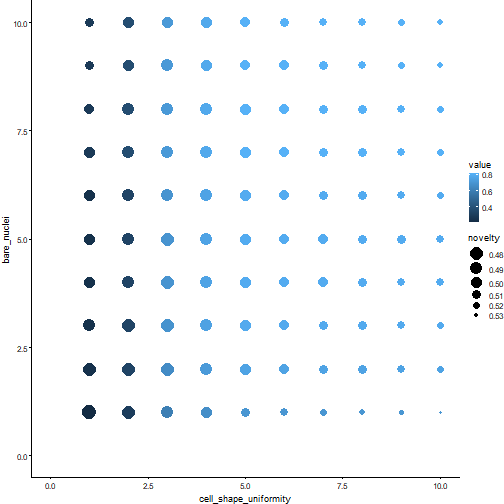The partial dependence plot above shows the cell_shape_uniformity along the x-axis and bare_nuclei along the y-axis. Higher intensities are associated with a higher expected probability of malignancy. The figure thus indicates that higher values of cell_shape_uniformity clearly affect the predicted malignancy probability. Higher values of bare_nuclei also lead to higher malignancy probabilities, but the effect is less pronounced. The average novelty score of the underlying instances determines the size of each point.

It also possible to anchor 2D partial dependence plots:

plots <- familiar::plot_ice(object=binomial_model,
data=binomial_data,
features=c("cell_shape_uniformity", "bare_nuclei"),
anchor_values=list("cell_shape_uniformity"=10.0,
"bare_nuclei"=10.0),
show_novelty=FALSE)

grid::grid.newpage()
grid::grid.draw(plots[])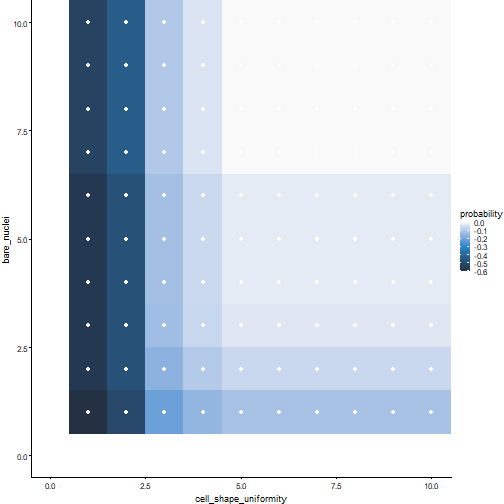We disabled novelty (show_novelty=FALSE) for plotting the 2D anchored plot. This changes the appearance of the plot, which now consists of coloured rectangles. The white dots show the position at which familiar samples the feature values.

Familiar automatically determines the values at which numerical features are sampled based on their distribution in the development dataset. Such values can also be manually set using the feature_x_range and feature_y_range arguments. Whereas for the breast cancer dataset the features are linearly sampled, for the nodes feature in the colon cancer dataset this is not the case:

plots <- familiar::plot_ice(object=survival_model,
data=survival_data,
features=c("nodes", "rx"),
evaluation_times=1000,
show_novelty=FALSE)

grid::grid.newpage()
grid::grid.draw(plots[])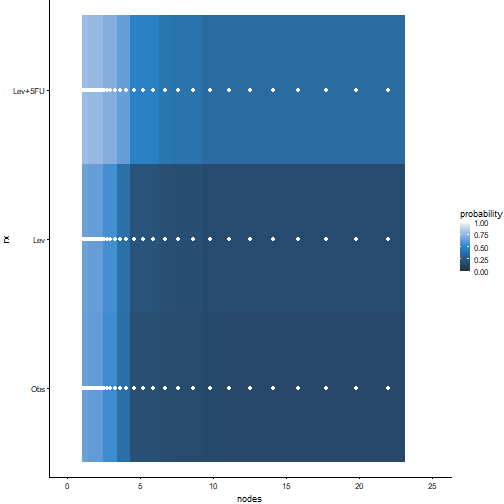Here we see that the number of nodes was not sampled linearly. Survival probability at 1000 days drops with an increase in nodes. Moreover, the model predicts that patients in the Levamisole Lev and observation Obs arms have lower survival probabilities overall.

# Feature and sample similarity

Highly similar features can carry redundant information for a model. This can be visualised using similarity heatmaps:

plots <- familiar::plot_feature_similarity(object=binomial_model,
data=binomial_data,
feature_similarity_metric="spearman")

grid::grid.newpage()
grid::grid.draw(plots[])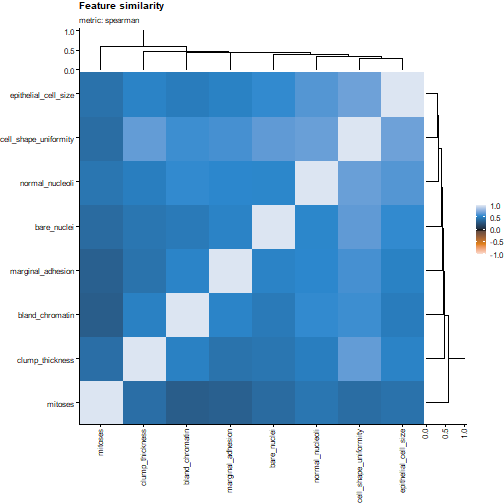The heatmap displays Spearman’s correlation coefficient between the different features. The features are also ordered by similarity, so that clusters of similar features can be identified. Note that the dendrograms depict cluster distance, i.e. $$1-|\rho|$$ for Spearman’s correlation coefficient.

It is also possible to view the sample clustering. This can be used to identify if samples are readily stratified into clusters, which may or may not correlate to the endpoint of interest. For the breast cancer dataset, this yields the following heatmap:

plots <- familiar::plot_sample_clustering(object=binomial_model,
data=binomial_data,
feature_similarity_metric="spearman")

grid::grid.newpage()
grid::grid.draw(plots[])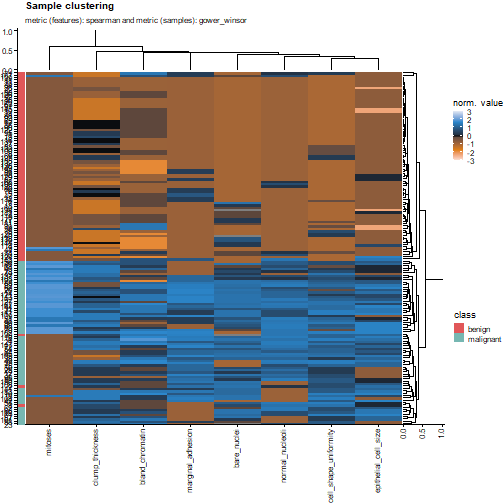As may be observed, samples in the breast cancer dataset form two main clusters that correspond closely to cell malignancy. The top dendrogram is the same as for feature clustering, as it should be. The right dendrogram is formed after computing Gower’s distance between samples (by default), and shows the division of samples into two main clusters. The heatmap itself shows normalised values for the features, based on normalisation and transformation parameters obtained during model development.

Normalisation options can be altered by changing the show_normalised_data argument. In the breast cancer dataset all features have the same range, so we can also show the data directly:

plots <- familiar::plot_sample_clustering(object=binomial_model,
data=binomial_data,
feature_similarity_metric="spearman",
show_normalised_data="none")

grid::grid.newpage()
grid::grid.draw(plots[])`